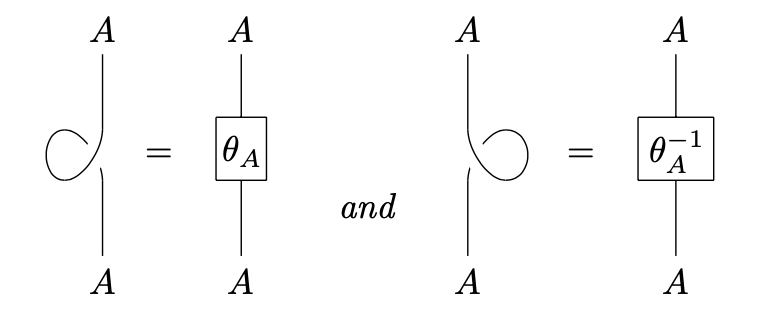# nLab balanced monoidal category

Balanced monoidal categories

### Context

#### Monoidal categories

monoidal categories

With braiding

With duals for objects

With duals for morphisms

With traces

Closed structure

Special sorts of products

Semisimplicity

Morphisms

Internal monoids

Examples

Theorems

In higher category theory

# Balanced monoidal categories

## Definition

A twist, or balance, in a braided monoidal category $\mathscr{C}$ is a natural isomorphism $\theta$ from the identity functor on $\mathscr{C}$ to itself satisfying the following compatibility condition with the braiding:

$\theta_{A\otimes B}=\beta_{B,A}\circ \beta_{A,B}\circ (\theta_A\otimes \theta_B),\,\, \forall A,B\in\mathscr{C}$

where $\beta$ is the braiding on $\mathscr{C}$. A balanced monoidal category is a braided monoidal category equipped with such a balance. Equivalently, a balanced monoidal category can be described as a braided pivotal category.

Balanced monoidal categories should not be confused with the other unrelated notation of a balanced category.

## Properties

Every symmetric monoidal category is balanced in a canonical way. In fact, the identity natural transformation on the identity functor of $\mathscr{C}$ is a balance on $\mathscr{C}$. In this way, the twist can be seen as a way of “controlling” the non-symmetric behavior of the braiding.

In the language of string diagrams, the balancing is represented by a 360-degree twist:This definition is taken from Jeff Egger (Appendix C), but the original definition can be found in chapter 4 of this paper by Joyal and Street:

• A. Joyal, R. Street, The geometry of tensor calculus I, Adv. Math. 88(1991), no. 1, 55–112, doi.